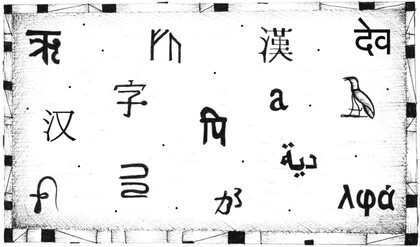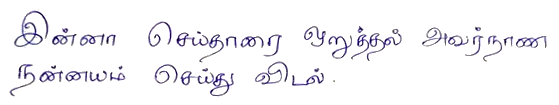## 五、高阶函数

Tzu-li and Tzu-ssu were boasting about the size of their latest programs. ‘Two-hundred thousand lines,’ said Tzu-li, ‘not counting comments!’ Tzu-ssu responded, ‘Pssh, mine is almost a million lines already.’ Master Yuan-Ma said, ‘My best program has five hundred lines.’ Hearing this, Tzu-li and Tzu-ssu were enlightened.

Master Yuan-Ma，《The Book of Programming》

There are two ways of constructing a software design: One way is to make it so simple that there are obviously no deficiencies, and the other way is to make it so complicated that there are no obvious deficiencies.

C.A.R. Hoare，1980 ACM Turing Award Lecture``````let total = 0, count = 1;
while (count <= 10) {
total += count;
count += 1;
}
console.log(total);
``````

``````console.log(sum(range(1, 10)));
``````

`sum``range`这两个函数定义的操作当然会包含循环、计数和其他一些操作。但相比于将这些代码直接写到一起，这种表述方式更为简单，同时也易于避免错误。

## 重复的抽象

``````for (let i = 0; i < 10; i++) {
console.log(i);
}
``````

``````function repeatLog(n) {
for (let i = 0; i < n; i++) {
console.log(i);
}
}
``````

``````function repeat(n, action) {
for (let i = 0; i < n; i++) {
action(i);
}
}

repeat(3, console.log);
// → 0
// → 1
// → 2
``````

``````let labels = [];
repeat(5, i => {
labels.push(`Unit \${i + 1}`);
});
console.log(labels);
// → ["Unit 1", "Unit 2", "Unit 3", "Unit 4", "Unit 5"]
``````

## 高阶函数

``````function greaterThan(n) {
return m => m > n;
}
let greaterThan10 = greaterThan(10);
console.log(greaterThan10(11));
// → true
``````

``````function noisy(f) {
return (...args) => {
console.log("calling with", args);
let result = f(...args);
console.log("called with", args, ", returned", result);
return result;
};
}
noisy(Math.min)(3, 2, 1);
// → calling with [3, 2, 1]
// → called with [3, 2, 1] , returned 1
``````

``````function unless(test, then) {
if (!test) then();
}
repeat(3, n => {
unless(n % 2 == 1, () => {
console.log(n, "is even");
});
});
// → 0 is even
// → 2 is even
``````

``````["A", "B"].forEach(l => console.log(l));
// → A
// → B
``````

## 脚本数据集``````{
name: "Coptic",
ranges: [[994, 1008], [11392, 11508], [11513, 11520]],
direction: "ltr",
year: -200,
living: false,
}
``````

`ranges`属性包含 Unicode 字符范围数组，每个数组都有两元素，包含下限和上限。 这些范围内的任何字符码都会分配给脚本。 下限是包括的（代码 994 是一个科普特字符），并且上限排除在外（代码 1008 不是）。

## 数组过滤

``````function filter(array, test) {
let passed = [];
for (let element of array) {
if (test(element)) {
passed.push(element);
}
}
return passed;
}

console.log(filter(SCRIPTS, script => script.living));
// → [{name: "Adlam", …}, …]
``````

`forEach`一样，`filter`函数也是标准的数组方法。本例中定义的函数只是用于展示内部实现原理。今后我们会使用以下方法来过滤数据：

``````console.log(SCRIPTS.filter(s => s.direction == "ttb"));
// → [{name: "Mongolian", …}, …]
``````

## 使用`map`函数转换数组

`map`方法对数组中的每个元素调用函数，然后利用返回值来构建一个新的数组，实现转换数组的操作。新建数组的长度与输入的数组一致，但其中的内容却通过对每个元素调用的函数“映射”成新的形式。

``````function map(array, transform) {
let mapped = [];
for (let element of array) {
mapped.push(transform(element));
}
return mapped;
}

let rtlScripts = SCRIPTS.filter(s => s.direction == "rtl");
console.log(map(rtlScripts, s => s.name));
// → ["Adlam", "Arabic", "Imperial Aramaic", …]
``````

`forEach``filter`一样，`map`也是标准的数组方法。

## 使用`reduce`汇总数据

`reduce`函数包含三个参数：数组、执行合并操作的函数和初始值。该函数没有`filter``map`那样直观，所以仔细看看：

``````function reduce(array, combine, start) {
let current = start;
for (let element of array) {
current = combine(current, element);
}
return current;
}

console.log(reduce([1, 2, 3, 4], (a, b) => a + b, 0));
// → 10
``````

``````console.log([1, 2, 3, 4].reduce((a, b) => a + b));
// → 10
``````

``````function characterCount(script) {
return script.ranges.reduce((count, [from, to]) => {
return count + (to - from);
}, 0);
}

console.log(SCRIPTS.reduce((a, b) => {
return characterCount(a) < characterCount(b) ? b : a;
}));
// → {name: "Han", …}
``````

`characterCount`函数通过累加范围的大小，来减少分配给脚本的范围。 请注意归约器函数的参数列表中使用的解构。 `reduce'的第二次调用通过重复比较两个脚本并返回更大的脚本，使用它来查找最大的脚本。

Unicode 标准分配了超过 89,000 个字符给汉字脚本，它成为数据集中迄今为止最大的书写系统。 汉字是一种（有时）用于中文，日文和韩文的文字。 这些语言共享很多字符，尽管他们倾向于以不同的方式写它们。 （基于美国的）Unicode 联盟决定将它们看做一个单独的书写系统来保存字符码。 这被称为中日韩越统一表意文字（Han unification），并且仍然使一些人非常生气。

## 可组合性

``````let biggest = null;
for (let script of SCRIPTS) {
if (biggest == null ||
characterCount(biggest) < characterCount(script)) {
biggest = script;
}
}
console.log(biggest);
// → {name: "Han", …}
``````

``````function average(array) {
return array.reduce((a, b) => a + b) / array.length;
}

console.log(Math.round(average(
SCRIPTS.filter(s => s.living).map(s => s.year))));
// → 1185
console.log(Math.round(average(
SCRIPTS.filter(s => !s.living).map(s => s.year))));
// → 209
``````

``````let total = 0, count = 0;
for (let script of SCRIPTS) {
if (script.living) {
total += script.year;
count += 1;
}
}
console.log(Math.round(total / count));
// → 1185
``````

## 字符串和字符码

``````function characterScript(code) {
for (let script of SCRIPTS) {
if (script.ranges.some(([from, to]) => {
return code >= from && code < to;
})) {
return script;
}
}
return null;
}

console.log(characterScript(121));
// → {name: "Latin", …}
``````

`some`方法是另一个高阶函数。 它需要一个测试函数，并告诉你该函数是否对数组中的任何元素返回`true`

``````// Two emoji characters, horse and shoe
let horseShoe = "\ud83d\udc34\ud83d\udc5f";
console.log(horseShoe.length);
// → 4
console.log(horseShoe);
// → (Invalid half-character)
console.log(horseShoe.charCodeAt(0));
// → 55357 (Code of the half-character)
console.log(horseShoe.codePointAt(0));
// → 128052 (Actual code for horse emoji)
``````

JavaScript的`charCodeAt`方法为你提供了一个代码单元，而不是一个完整的字符代码。 稍后添加的`codePointAt`方法确实提供了完整的 Unicode 字符。 所以我们可以使用它从字符串中获取字符。 但传递给`codePointAt`的参数仍然是代码单元序列的索引。 因此，要运行字符串中的所有字符，我们仍然需要处理一个字符占用一个还是两个代码单元的问题。

``````let roseDragon = "\ud83c\udf45\ud83d\udc09";
for (let char of roseDragon) {
console.log(char);
// → (emoji rose)
// → (emoji dragon)
``````

## 识别文本

``````function countBy(items, groupName) {
let counts = [];
for (let item of items) {
let name = groupName(item);
let known = counts.findIndex(c => c.name == name);
if (known == -1) {
counts.push({name, count: 1});
} else {
counts[known].count++;
}
}
return counts;
}

console.log(countBy([1, 2, 3, 4, 5], n => n > 2));
// → [{name: false, count: 2}, {name: true, count: 3}]
``````

`countBy`函数需要一个集合（我们可以用`for/of`来遍历的任何东西）以及一个函数，它计算给定元素的组名。 它返回一个对象数组，每个对象命名一个组，并告诉你该组中找到的元素数量。

``````function textScripts(text) {
let scripts = countBy(text, char => {
let script = characterScript(char.codePointAt(0));
return script ? script.name : "none";
}).filter(({name}) => name != "none");

let total = scripts.reduce((n, {count}) => n + count, 0);
if (total == 0) return "No scripts found";

return scripts.map(({name, count}) => {
return `\${Math.round(count * 100 / total)}% \${name}`;
}).join(", ");
}

console.log(textScripts('英国的狗说"woof", 俄罗斯的狗说"тяв"'));
// → 61% Han, 22% Latin, 17% Cyrillic
``````

## 习题

### 展开

``````let arrays = [[1, 2, 3], [4, 5], ];
// → [1, 2, 3, 4, 5, 6]
``````

### 你自己的循环

``````// Your code here.

loop(3, n => n > 0, n => n - 1, console.log);
// → 3
// → 2
// → 1
``````

### `every`

`every`实现为一个函数，接受一个数组和一个谓词函数作为参数。编写两个版本，一个使用循环，另一个使用`some`方法。

``````function every(array, test) {